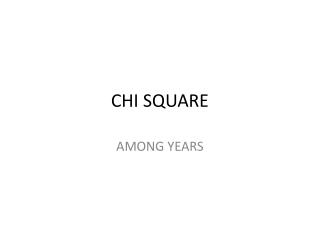Download PresentationCHI SQUARE

# CHI SQUARE

Download Presentation## CHI SQUARE

- - - - - - - - - - - - - - - - - - - - - - - - - - - E N D - - - - - - - - - - - - - - - - - - - - - - - - - - -
##### Presentation Transcript

1. CHI SQUARE AMONG YEARS

2. RESEARCH QUESTION • Is there any relationship between Emotional Intelligence(EI) level with the year of studies for year 1, year 2 and year 3 of biomedical students?

3. HYPOTHESIS • Null hypothesis : n1 = n2 = n3 The Emotional Intelligence (EI) for Year 1, Year 2 and Year 3 is the same. • Alternative hypothesis : n1 ≠ n2 ≠ n3 The Emotional Intelligence (EI) for Year 1, Year 2 and Year 3 is different.

4. CHI-SQUARE TEST • Case processing summary The case summary processing indicates that the data from 133 cases were included in these analyses.

5. YEAR EI CROSSTABULATION

6. From the table, the value of count of year 1 students that have moderate Emotional Intelligence is 17 and the percentage within year is 65.4%. For year 2, the count is 39 with 79.6% for the percentage withing year and 86.2% for year 3 with the count of 50 students. • From the table also, the value of count for year 1 students that have high Emotional Intelligence is 9 and the percentage within year is 34.6%. While, the value of count of year 2 students is 10 with 20.4% and 11.8% for the year 3 students with the value of count of 8.

7. CHI SQUARE TEST • The Pearson Chi-square is not statistically significant. This is because the Asymp. Sig. is 0.090 which is more than the α = 0.05. • We can conclude that the year of the studies forbiomedical science students is not related to the Emotional Intelligence level. • We do not reject null hypothesis.

8. CONCLUSION • A Pearson’s chi-square test of contingencies (with α = 0.05) was used to evaluate whether year of studies of the biomedical students is related to the Emotional Intelligence level or not. • The chi-square test was not statistically significant, p = 0.090. Year of studies of the students is not related to the Emotional Intelligence level.